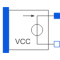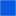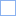# G_VCC_POLY

Polynomial voltage controlled current source, like SPICE2# Information

This information is part of the Modelica Standard Library maintained by the Modelica Association.

The polynomial source is a SPICE2 model, which is also known in other SPICE derivatives.

Nonlinear voltage controlled current source. The right port current at pin p2 (=p2.i) is controlled by the left port vector of voltages at the pin vector pc[:] via

`    p2.i = f(pc.v - pc.v, pc.v - pc.v,...)`

The controlling port (left) current vector is zero.

f is a polynomial in N variables s1...sN of the following form with M+1 coefficients a0, a1, a2,...aM.

```f = a0 +
a1s1 + a2s2 + ... + aNsN +
a(N+1)s1² + a(N+2)s1s2 + ... + a(.)s1sN +
a(.)s2² + a(.)s2s3 + ... + a(.)s2sN +
a(.)s3² + s3s4 + ... + a(.)s4sN +
... +
a(.)sN² +
a(.)s1³ + a(.)s1²s2 + a(.)s1²s3 + ... + a(.)s1²sN +
a(.)s1s2² + a(.)s1s2s3 + ... + a(.)s1s2sN +
... +
a(.)sN³ + ... ```

The Coefficients a(.) are counted in this order. Reaching M, the particular sum is canceled.

In connection with the VCC, s1...sN are the voltages of the controlling side: s1=pc.v - pc.v, s2=pc.v - pc.v, s3=...

The corresponding SPICE description of the VCC polynomial source is the following:

`    Gname A1 A2 POLY(N) E11 E21 ... E1N E2N P0 P1...`

where Gname is the name of the instance, A1 and A2 are the nodes between them the current source is arranged, whose current is calculated,

N is the number of the controlling voltages, E11 E12 ... E1N E2N are pairs of nodes between them the controlling voltages

are gripped, and P0, P1... are the coefficients that are called a0, a1, ... aM in the description of the polynomial f above.

To describe the SPICE line in Modelica, the following explanation would be useful:

```Gname -> G_VCC_POLY name
A1, A2 -> pins name.p2, name.p1
N -> parameter N
E11 -> name.pc
E12 -> name.pc
...
E1N -> name.pc[N]
E2N -> name.pc[N-1]
P0, P1 -> polynomial coefficients name.coeff(coeff={P0,P1,...}) ```

# Parameters (2)

N Value: 1 Type: Integer Description: Number of controlling voltages Value: {1} Type: Real[:] Description: Coefficients of polynomial

# Connectors (3)

p nType: PositivePin Description: Positive pin of the right port (potential p2.v > n2.v for positive voltage drop v2)Type: NegativePin Description: Negative pin of the right portType: PositivePin[2 * N] Description: Pin vector of controlling pins